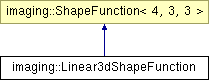# imaging::Linear3dShapeFunction Class Reference [Finite Element Module]

Linear shape function on the tetrahedra. More...

`#include <ShapeFunction.hpp>`

Inheritance diagram for imaging::Linear3dShapeFunction:List of all members.

## Public Member Functions

float_t value (size_t node_index, const ublas::fixed_vector< float_t, 3 > &in) const
ublas::fixed_vector< float_t, 3 > & gradient (size_t node_index, const ublas::fixed_vector< float_t, 3 > &in, ublas::fixed_vector< float_t, 3 > &out) const

## Static Public Member Functions

static size_t face_node (size_t face, size_t node)

## Detailed Description

Linear shape function on the tetrahedra.

Linear shape function on the tetrahedra determined by the vertices (0, 0, 0), (1, 0, 0), (0, 1, 0), (0, 0, 1) (i.e. 4 element nodes). You will rarely use this class directly, but might plug it into your own FE traits class as shape_function_t.

## Member Function Documentation

 size_t imaging::Linear3dShapeFunction::face_node ( size_t face, size_t node ) ` [static]`

Returns the node index (on the element) of the node determined by face_index and face_node_index (on the boundary reference element). E.g. the vertex 1 (there are only vertices 0 and 1 on the boundary reference element) on the face 2 of the square reference element (there are 4 faces with indices from zero to 3) is mapped to vertex 3 on the reference element.

Reimplemented from imaging::ShapeFunction< 4, 3, 3 >.

 float_t imaging::Linear3dShapeFunction::value ( size_t node_index, const ublas::fixed_vector< float_t, 3 > & in ) const

Returns the value of the shape function corresponding to node_index at in.

 ublas::fixed_vector< float_t, 3 > & imaging::Linear3dShapeFunction::gradient ( size_t node_index, const ublas::fixed_vector< float_t, 3 > & in, ublas::fixed_vector< float_t, 3 > & out ) const

Computes the gradient of the shape function corresponding to node_index at in and stores the result in out.

The documentation for this class was generated from the following files:

Generated on Tue Feb 10 10:01:31 2009 for imaging2 by1.5.5